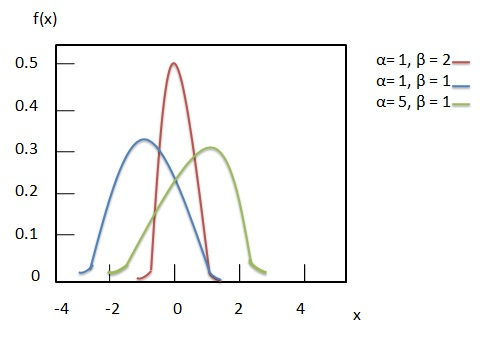# Statistics - Log Gamma Distribution

Log Gamma Distribution is a probability density function with positive shape parameters ${\alpha, \beta }$ and location parameter ${ \mu }$. It is defined by following formula.

## Formula

${ f(x) = \frac{e^{\beta x}e^{\frac{-e^x}{\alpha}}}{ \alpha^\beta \Gamma(\beta)} \\[7pt] \, where -\infty \gt x \lt \infty }$

Where −

• ${\alpha}$ = positive shape parameter.

• ${\beta}$ = positive shape parameter.

• ${x}$ = random variable.

Following diagram shows the probability density function with three different parameter combinations.